## Selina Concise Mathematics Class 6 ICSE Solutions Chapter 12 Proportion (Including Word Problems)

Selina Publishers Concise Mathematics Class 6 ICSE Solutions Chapter 12 Proportion (Including Word Problems)

APlusTopper.com provides step by step solutions for Selina Concise ICSE Solutions for Class 6 Mathematics. You can download the Selina Concise Mathematics ICSE Solutions for Class 6 with Free PDF download option. Selina Publishers Concise Mathematics for Class 6 ICSE Solutions all questions are solved and explained by expert mathematic teachers as per ICSE board guidelines.

### Proportion Exercise 12A – Selina Concise Mathematics Class 6 ICSE Solutions

Question 1.
In each of the following, check whether or not the given ratios form a proportion :
(i) 8 : 16 and 12 : 15
(ii) 16 : 28 and 24 : 42
(iii) 12 ÷ 3 and 8 ÷ 2
(iv) 25 : 40 and 20 : 32
(v) $$\frac { 15 }{ 18 } and \frac { 10 }{ 12 }$$
(vi) $$\frac { 7 }{ 8 }$$ and 14 : 16
Solution: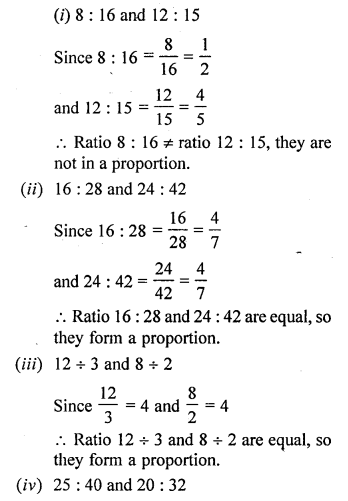Question 2.
Find the value of x in .each of the following proportions :
(i) x : 4 = 6 : 8
(ii) 14 : x = 7 : 9
(iii) 4 : 6 = x : 18
(iv) 8 : 10 = x : 25
(v) 5 : 15 = 4 : x
(vi) 16 : 24 = 6 : x
Solution: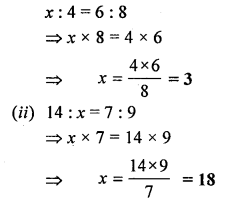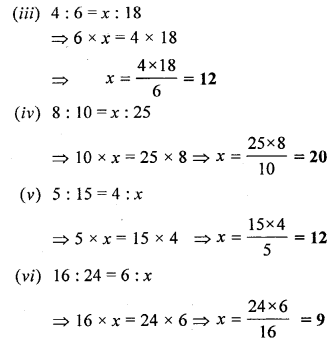Question 3.
Find the value of x so that the given four numbers are in proportion :
(i) x, 6, 10 and 15
(ii) x, 4, 15 and 30
(iii) 2, x, 10 and 25
(iv) 4, x, 6 and 18
(v) 9, 12, x and 8
(vi) 4, 10, 36 and x
(vii) 7, 21, x and 45
(viii) 6, 8, 12 and x.
Solution: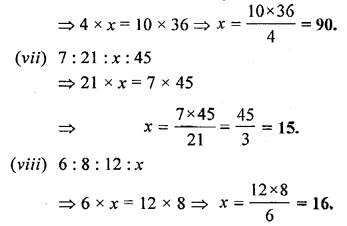Question 4.
The first, second and the fourth terms of a proportion are 6, 18 and 75, respectively. Find its third term.
Solution:
Let the third term = x
6 : 18 : : x : 75
= 18 x x = 6 x 75
x = $$\frac { 6\times 75 }{ 18 } =\frac { 75 }{ 3 }$$ = 25
The third term of proportion is 25

Question 5.
Find the second term of the proportion whose first, third and fourth terms are 9, 8 and 24 respectively.
Solution:
Let the second term = x
9 : x : : 8 : 24
=> x x 8 = 24 x 9
x = $$\frac { 24\times 9 }{ 8 }$$ = 3 x 9 = 27
The second term of proportion = 27

Question 6.
Find the fourth term of the proportion whose first, second and third terms are 18, 27, and 32 respectively.
Solution:
Let the fourth term = x
18 : 27 : : 32 : x
=> 18 x x = 27 x 32
=> x = $$\frac { 27\times 32 }{ 18 }$$ = 3 x 16 = 48
Fourth term = 48

Question 7.
The ratio of the length and the width of a school ground is 5 : 2. Find the length, if the width is 40 metres.
Solution:
Let the length = x m,
width = 40 m
The ratio of length to width = x : 40
as per given statement 5 : 2 = x : 40
=> 2 x x = 40 x 5
x = $$\frac { 40\times 5 }{ 2 }$$ = 20 x 5 = 100 m

Question 8.
The ratio of the sale of eggs on a Sunday and that of the whole week at a grocery shop was 2 : 9. If the total value of the sale of eggs in the same week was Rs 360, find the value of the sale of eggs that Sunday.
Solution:
Let, the sale of eggs on Sunday = x
Sale in week = Rs 360
According to question, 2 : 9 = x : 360
=> 9 x x = 360 x 2
x = $$\frac { 360\times 2 }{ 9 }$$ = Rs 80
Sale on Sunday = Rs 80

Question 9.
The ratio of copper and zinc in an alloy is 9 : 8. If the weight of zinc, in the alloy, is 9.6 kg ; find the weight of copper in the alloy.
Solution:
Let the weight of copper = x kg
Weight of zinc = 9.6 kg.
According to question,
9 : 8 = x : 9.6
=> 8 x x = 9 x 9.6
=> x = $$\frac { 9\times 9.6 }{ 8 }$$ = 9 x 1.2 = 10.8 kg.
Weight of cooper in alloy = 10.8

Question 10.
The ratio of the number of girls to the number of boys in a school is 2 : 5. If the number of boys is 225 ; find:
(i) the number of girls in the school.
(ii) the number of students in the school.
Solution:
Let, the number of girls in school = x
Number of boys in school = 225
According to question 2 : 5 = x : 225
=> 5 x x = 2 x 225
x = $$\frac { 2\times 225 }{ 5 }$$ = 2 x 45 = 90
Number of girls in school = 90
Total number of student in the school = (number of boys + number of girls) = (225 + 90) = 315

Question 11.
In a class, one out of every 5 students pass. If there are 225 students in all the sections of a class, find how many pass ?
Solution:
Total number of students in all sections = 225
Given, One of every five students pass
Total students pass = 225 x $$\frac { 1 }{ 5 }$$ = 45 studetns

Question 12.
Make set of all possible proportions from the numbers 15, 18, 35 and 42.
Solution:
The possible proportions that can be made from the numbers 15, 18, 35 and 42 are
(i) 15 : 35 :: 18 : 42
(ii) 42 : 18 :: 35 : 15
(iii) 42 : 35 :: 18 : 15
(iv) 15 : 18 :: 35 : 42

### Proportion Exercise 12B – Selina Concise Mathematics Class 6 ICSE Solutions

Question 1.
If x, y and z are in continued proportion, then which of the following is true :
(i) x : y = x : z
(ii) x : x = z : y
(iii) x : y = y : z
(iv) y : x = y : z
Solution:
(iii) x : y = y : z

Question 2.
Which of the following numbers are in continued proportion :
(i) 3, 6 and 15
(ii) 15, 45 and 48
(iii) 6, 12 and 24
(iv) 12, 18 and 27
Solution:
(iii) and (iv)

Question 3.
Find the mean proportion between
(i) 3 and 27
(ii) 0.06 and 0.96
Solution:Question 4.
Find the third proportional to :
(i) 36, 18
(ii) 5.25, 7
(iii) ₹ 1.60, ₹ 0.40
Solution: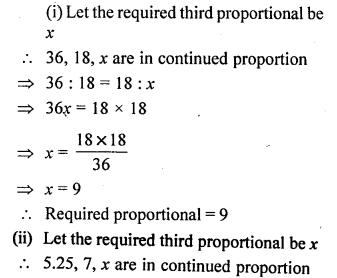=> x = 0.1

Question 5.
The ratio between 7 and 5 is same as the ratio between ₹ x and ₹ 20.50 ; find the value of x.
Solution:
Since, It is given that the ratio between 7 and 5 is same as the ratio between ₹ x and ₹ 20.50Question 6.
If (4x + 3y) : (3x + 5y) = 6 : 7, find :
(i) x : y
(ii) x, if y = 10
(iii) y, if x = 27
Solution: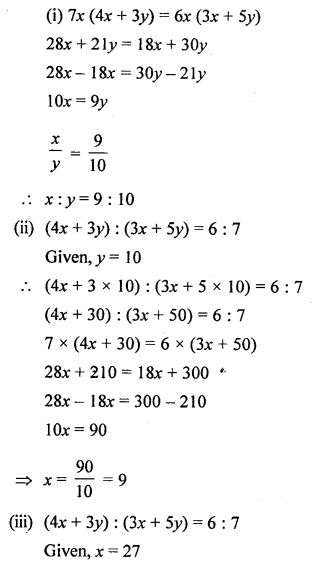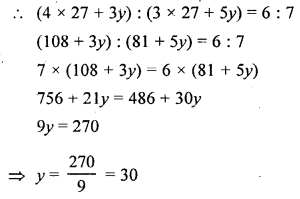Question 7.
If $$\frac { 2y+5x }{ 3y-5x } =2\frac { 1 }{ 2 }$$, find:
(i) x : y
(ii) x, if y = 70
(iii) y, if x = 33
Solution: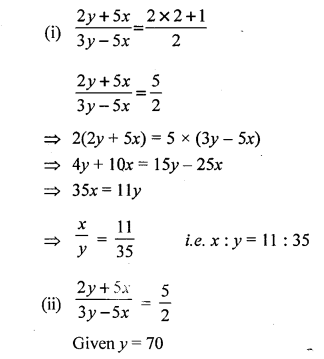### Proportion Exercise 12C – Selina Concise Mathematics Class 6 ICSE Solutions

Question 1.
Are the following numbers in proportion:
(i) 32, 40, 48 and 60 ?
(ii) 12,15,18 and 20 ?
Solution:
(i) 32, 40, 48 and 60 are in proportion
if 32 : 40 = 48 : 60
if 32 x 60 = 40 x 48
$$\left\{ \frac { a }{ b } =\frac { c }{ d } \Longrightarrow \quad ad=bc \right\}$$
if 1920 = 1920
Which is true.
32, 40, 48 and 60 are in proportion
(ii) 12, 15, 18 and 20 are in proportion
if 12 : 15 = 18 : 20
if 12 x 20 = 15 x 18 {ad = bc}
if 240 = 270
which is not true.
12, 15, 18 and 20 are not in proportion.

Question 2.
Find the value of x in each of the following such that the given numbers are in proportion.
(i) 14, 42, x and 75
(ii) 45, 135, 90 and x
Solution:
14, 42, x and 75 are in proportion
$$\frac { 14 }{ 42 } =\frac { x }{ 75 }$$
=> 14 x 75 =x x 42Question 3.
The costs of two articles are in the ratio 7 : 4. If the cost of the first article is Rs. 2,800 ; find the cost of the second article.
Solution:
Ratio in the cost of two articles = 7 : 4
Cost of first article = Rs. 2800
Let cost of the second article = x
7 : 4 = 2800 : x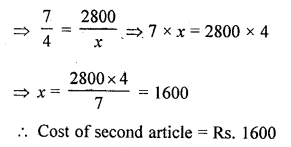Question 4.
The ratio of the length and the width of a rectangular sheet of paper is 8 : 5. If the width of the sheet is 17.5 cm; find the length.
Solution:
Let length of sheet = x cm
Ratio in length and breadth = 8 : 5
and width = 17.5 cm
8 : 5 = x : 17.5Length of sheet = 28 cm

Question 5.
The ages of A and B are in the ratio 6 : 5. If A’s age is 18 years, find the age of B.
Solution:
Ratio in the ages of A and B = 6 : 5
A’s age = 18 years
Let B’s age = x years
6 : 5 = 18 : x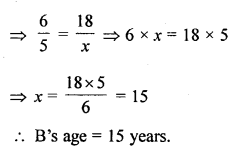Question 6.
A sum of Rs. 10, 500 is divided among A, B and C in the ratio 5 : 6 : 4. Find the share of each.
Solution:
Total amount = Rs. 10, 500
Ratio in A, B, and C = 5 : 6 : 4
Sum of ratio = 5 + 6 + 4 = 15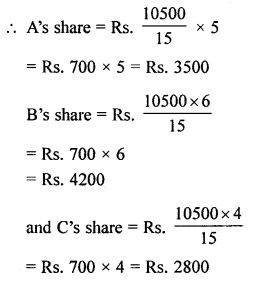Question 7.
Do the ratios 15 cm to 2 m and 10 sec to 3 minutes form a proportion ?
Solution:
15 cm : 2 m : : 10 sec : 3 min
15 cm : 2 x 100 cm :: 10 sec : 30 x 60 sec
15 : 200 :: 10 : 1800
3 : 40 :: 1 : 180
No, they donot form a proportion

Question 8.
Do the ratios 2 kg : 80 kg and 25 g : 625 g form a proportion ?
Solution:
2 kg : 80 kg : : 25 g : 625 g
2 : 80 :: 25 : 625
1 : 40 :: 1 : 25
No, they donot form a proportion.

Question 9.
10 kg sugar cost ₹ 350. If x kg sugar of the same kind costs ₹ 175, find the value of x
Solution:
10 kg of sugar costs = ₹ 350
and x kg of sugar cost = ₹ 175
A.T.Q.
10 kg : x kg :: 350 : 175
=> 10 x 175 = 350 x x
=> 350x= 1750
=> x = $$\frac { 1750 }{ 350 }$$ = 5
Hence, 5 kg of sugar costs ₹ 175

Question 10.
The length of two ropes are in the ratio 7 : 5. Find the length of:
(i) shorter rope, if the longer one is 22.5 ni
(ii) longer rope, if the shorter is 9.8 m.
Solution:
Length of the ropes are in the ratio = 7 : 5
(i) Let the length of shorter rope = x
Length of longer rope = 22.5 m
A.T.Q.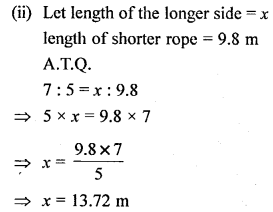Question 11.
If 4, x and 9 are in continued proportion, find the value of x.
Solution:
4, x and 9 are in continued proportion
=> 4 : x = x : 9
=> x2 = 9 x 4
=> x = √36
x = 6

Question 12.
If 25, 35 and x are in continued proportion, find the value of x.
Solution:
25, 35 and x are in continued proportion
=> 25 : 35 = 35 : x
=> 25 x x = 35 x 35
=> x = $$\frac { 35\times 35 }{ 25 }$$
=> x = 49